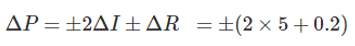Engineering Jobs   »   ELECTRICAL GATE QUIZ

# GATE’22 EE: Daily Practices Quiz 28-July-2021

Each question carries 2 marks
Negative marking: 0.66 mark

Total Questions: 05
Total marks: 10
Time: 12 min.

Q1. A power flow problem is solved by Newton-Raphson method in polar coordinate. The Jacobian size is 100×100. The system has 20 PV buses in addition to PQ buses and one slack bus. The total numbers of buses in the system are
(a) 63
(b) 61
(c) 64
(c) 65

Q2. A 25-bus power system has 5 generator buses and 20 load buses. For the load flow analysis using Newton Raphson method in polar coordinates, the size of the Jacobian matrix is ________.
(a) 48 x 48
(b) 25 x 25
(c) 28 x 28
(d) 44 x 44

Q3. For a circuit given that I = 2 ± 5 % A, R = 100 ± 0.2 % Ω the limiting error in the power dissipation I2 R in the resistor R is
(a) 1.2 %
(b) 5.2 %
(c) 10.2 %
(d) 25.2 %

Q4. A load is connected to a circuit. At the terminals to which the load is connected, R_Th = 10 Ω and V_Th = 40 V. The maximum power supplied to the load is
(a) 160 W
(b) 80 W
(c) 1 W
(d) 40 W

Q5. A dc shunt motor runs at a no-load speed of 1140 rpm. At full load, armature reaction weakens the main flux by 5% whereas the armature circuit voltage drops by 10%. The motor full-load speed in rpm is
(a) 1000
(b) 1203
(c) 1080
(d) 1200

SOLUTIONS

S1. Ans.(b)
Sol. ACQ: The Jacobian size is 100×100 & the system has 20 PV buses in which slack bus is not included. i.e., one slack bus is present apart from this.
If n=number of buses & m=PV or generator buses, then
∴ Size of Jacobian matrix=(2n-m-2)×(2n-m-2)
⇒100×100=(2n-20-2)×(2n-20-2)
∵(2n-20-2)=100
⇒n=(100+22)/2=61

S2. Ans.(d)
Sol. Given that 5 generator buses, we need to assume with in the 5 buses one bus as slack bus.
If n=number of buses & m=PV or generator buses, then
Size of Jacobian matrix=(2n-m-1)×(2n-m-1)
=(2×25-5-1)×(2×25-5-1)=44×44.

S3. Ans.(c)
Sol. Power dissipation P=I2RS4. Ans.(d)
Sol. Under maximum power transfer, R_L=R_Th and voltage across V_L=V_Th/2.
∴P_(max.)=(V_Th/2)²/R_Th =(40/2)²/10=40 W

S5. Ans.(c)
Sol. N α E/ϕ
∴N_2/N_1 =(E_2 ϕ_1)/(E_1 ϕ_2 )
∴N_2/N_1 =(0.9E_1×ϕ_1)/(E_1×0.95 ϕ_1 )
⇒N_2=1140×0.9/0.95=1080 rpm

Sharing is caring!